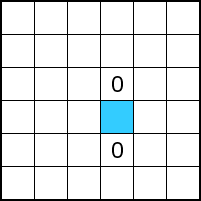# Tips

Find pairs

Because no more than two similar numbers next to or below each other are allowed, pairs can be supplementen with the other number. In the example below, both blue boxes should contain a zero.Avoid trios

If two cells contain the same number with an empty cell in between, this empty cell should contain the other number. The blue cell in the example below should contain a one, because otherwise a trio appears.Complete rows and columns

Each row and each column should contain an equal number of zeros and ones. If the required number of zeros is reached in a row or a column, the remaining cells should contain a one, and vice versa. In the example below, each row and each column should contain 3 ones and 3 zeros. This implies that the blue cell in the third row should be a one, and that the two blue cells in the third column should be a zero.Eliminate impossible combinations based on completed rows and columns

In the example below, there is a one and a zero missing in the lower column. If the one is filled in in the left blue cell and the zero in the right blue cell, a row similar to the second row is obtained. This is not allowed. Therefore a zero should be filled in in the left blue cell and a one in the right blue cell.Eliminate other impossible combinations

In the situation below there is a one and there are two zeros missing in the fourth row. If we fill in the one in the blue cell, we end up with three zeros in a row. Because this is not allowed, it follows that the blue cell should contain a zero.